# Calculus 2 : Comparing Series

## Example Questions

← Previous 1 3

### Example Question #1 : Comparing Series

We consider the series having the general term :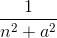Determine the nature of convergence of the series.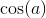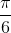The series is divergent.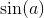The series is convergent.

The series is convergent.

Explanation:

We will use the integral test to prove this result.

We need to note the following:is positive, decreasing and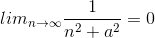.

By the integral test, we know that the series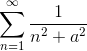is the integral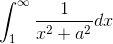.

We know that the above intgral is finite.

This means that the seriesis convergent.

### Example Question #2 : Comparing Series

We know that :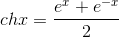and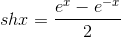We consider the series having the general term: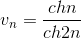Determine the nature of the series: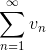The series is convergent.

It will stop converging after a certain number.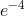The series is divergent.

The series is convergent.

Explanation:

We know that: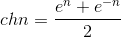and therefore we deduce :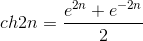We will use the Comparison Test with this problem. To do this we will look at the function in general form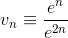We can do this since,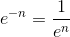and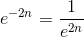approach zero as n approaches infinity. The limit of our function becomes,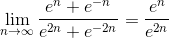This last part gives us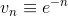.

Now we know that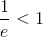and noting that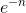is a geometric series that is convergent.

We deduce by the Comparison Test that the series

having general term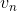is convergent.

### Example Question #3 : Comparing Series

We consider the following series: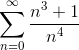Determine the nature of the convergence of the series.The series is divergent.The series is divergent.

Explanation:

We will use the comparison test to prove this result. We must note the following: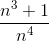is positive.

We have all natural numbers n: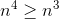, this implies that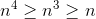.

Inverting we get :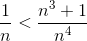Summing from 1 to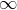, we have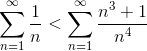We know that the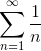is divergent. Therefore by the comparison test: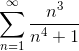is divergent

### Example Question #4 : Comparing Series

Determine the nature of convergence of the series having the general term: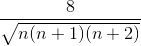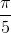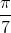The series is divergent.

The series is convergent.

The series is convergent.

Explanation:

We will use the Limit Comparison Test to establish this result.

We need to note that the following limit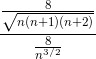goes to 1 as n goes to infinity.

Therefore the series have the same nature. They either converge or diverge at the same time.

We will focus on the series: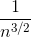.

We know that this series is convergent because it is a p-series. (Remember that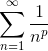converges if p>1 and we have p=3/2 which is greater that one in this case)

By the Limit Comparison Test, we deduce that the series is convergent, and that is what we needed to show.

### Example Question #1 : Comparing Series

Determine whether the following series is convergent or divergent.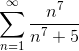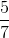This series is divergent.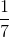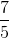This series is divergent.

Explanation:

To have a series that is convergent we must have that the general term of the series goes to 0 as n goes to.

We have the general term: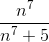therefore, we have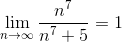.

This means that the general term does not go to 0 .

Therefore the series is divergent.

### Example Question #1 : Comparing Series

Determine the nature of the following series: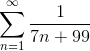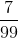The series is convergent.The series is divergent.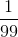The series is divergent.

Explanation:

We note first that the general term of the series is positive.

It is also decreasing and tends to 0 as n tends to. We will use the Integral Comparison Test to show this result.

Note the nature of the series is the same for the integral: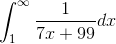This last intgral is divergent because it does not equal zero.

Therefore our series is divergent as well.

### Example Question #1 : Comparing Series

Determine the nature of the series having the general term: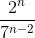The series is convergent.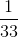The series is divergent.

The series is convergent.

Explanation:

We note first that we can write the general term as: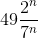and simplifying this term one more time, we have: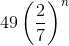We note that since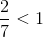,  this series is a geometric one which is convergent.

This is what we need to show here.

### Example Question #2 : Comparing Series

Determine whether the following series is convergent or divergent: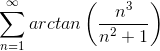This series is convergent.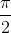This series is divergent.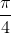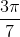This series is divergent.

Explanation:

We know that if a series is convergent, then its general term must go to 0 as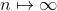.

We have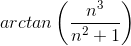is our general term in this case.

We have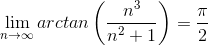.

Since the general term does not go to 0, the series is divergent.

### Example Question #9 : Comparing Series

Using the Limit Test, determine the nature of the series: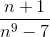The series is convergent.

The series is divergent.

The series is convergent.

Explanation:

We will use the Limit Comparison Test to study the nature of the series.

We note first that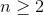, the series is positive.

We will compare the general term to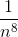We note that by letting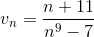and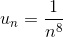, we have: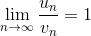.

Therefore the two series have the same nature, (they either converge or diverge at the same time).

We will use the Integral Test to deduce that the series having the general term:is convergent.

Note that we know that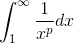is convergent if p>1 and in our case p=8 .

This shows that the series having general term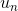is convergent.

By the Limit Test, the series having general termis convergent.

This shows that our series is convergent.

### Example Question #3 : Comparing Series

Determine the nature of the series having general term: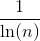whereThe series is convergent.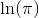The series is divergent.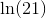The series is divergent.

Explanation:

We will use the Comparison Test to prove this result.

We need to note first that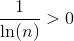for.

We know that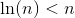, where.

Inverting the above inequality, we have: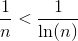.

Now we will use the Comparison Test.

We know that the series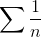is divergent.

Therefore,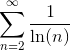is also divergent.

← Previous 1 3